Скачать презентацию Lecture 14 Queuing Systems Topics Basic

215f8ff2106c5f32b7fdedb6d4884566.ppt

• Количество слайдов: 45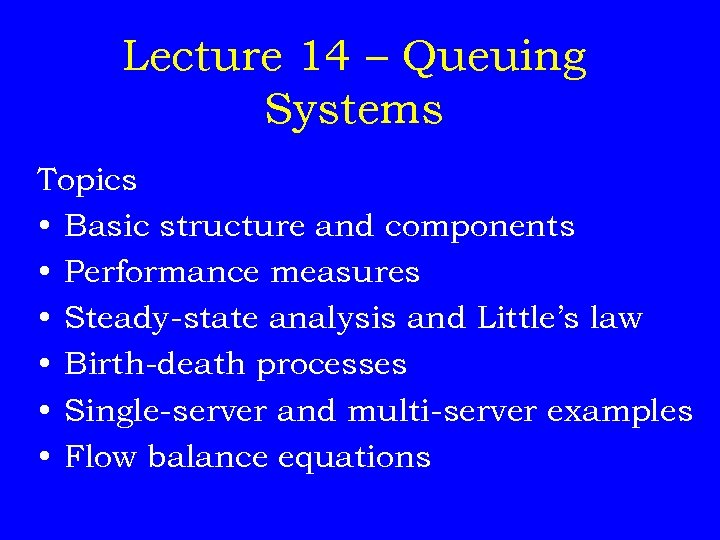Lecture 14 – Queuing Systems Topics • Basic structure and components • Performance measures • Steady-state analysis and Little’s law • Birth-death processes • Single-server and multi-server examples • Flow balance equations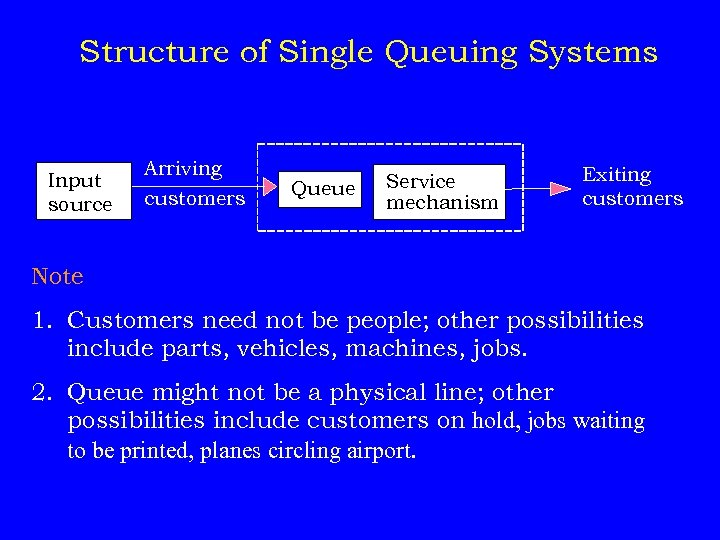Structure of Single Queuing Systems Input source Arriving customers Queue Service mechanism Exiting customers Note 1. Customers need not be people; other possibilities include parts, vehicles, machines, jobs. 2. Queue might not be a physical line; other possibilities include customers on hold, jobs waiting to be printed, planes circling airport.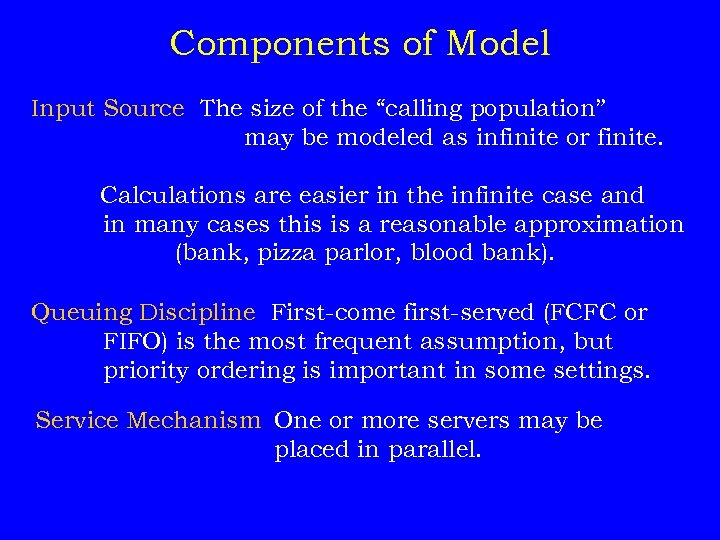Components of Model Input Source The size of the “calling population” may be modeled as infinite or finite. Calculations are easier in the infinite case and in many cases this is a reasonable approximation (bank, pizza parlor, blood bank). Queuing Discipline First-come first-served (FCFC or FIFO) is the most frequent assumption, but priority ordering is important in some settings. Service Mechanism One or more servers may be placed in parallel.Queuing Applications System Arrival Process Service Process Bank Customers Arrive Tellers serve customers Pizza parlor Orders are phoned in Orders are driven to customers Blood bank Pints of blood arrive via donation Patients use up pints of blood Shipyard Damaged ships sent Ships are repaired to shipyard for repair & return to sea Printers Jobs arrive from computers Documents are printed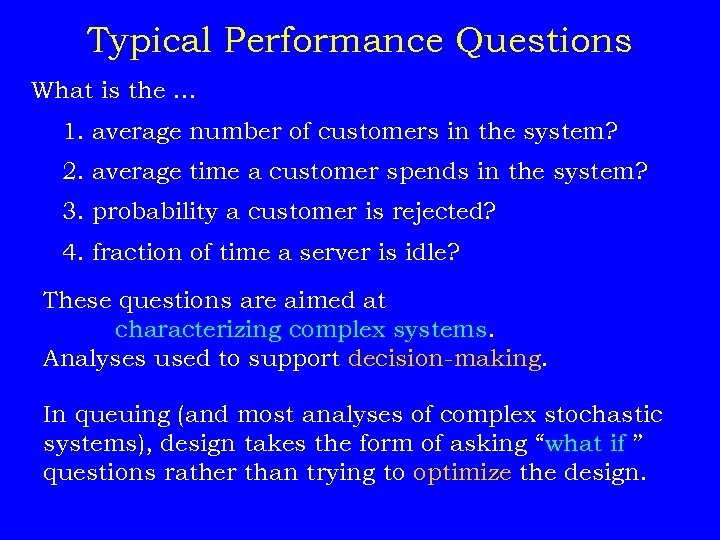Typical Performance Questions What is the. . . 1. average number of customers in the system? 2. average time a customer spends in the system? 3. probability a customer is rejected? 4. fraction of time a server is idle? These questions are aimed at characterizing complex systems. Analyses used to support decision-making. In queuing (and most analyses of complex stochastic systems), design takes the form of asking “what if ” questions rather than trying to optimize the design.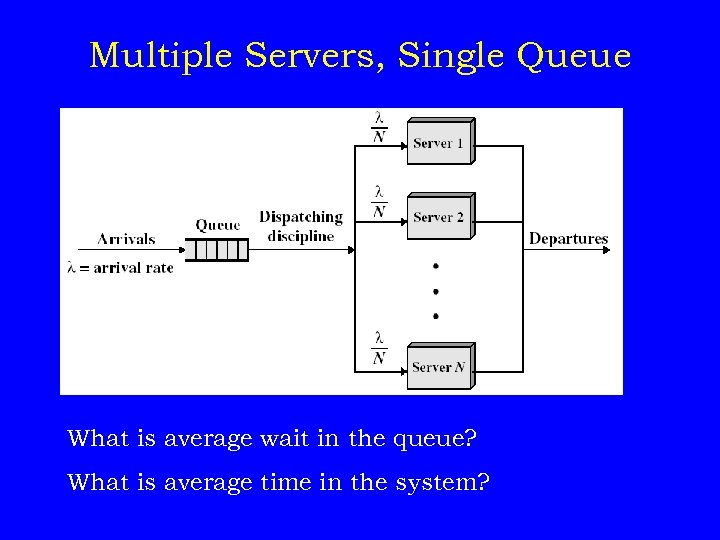Multiple Servers, Single Queue What is average wait in the queue? What is average time in the system?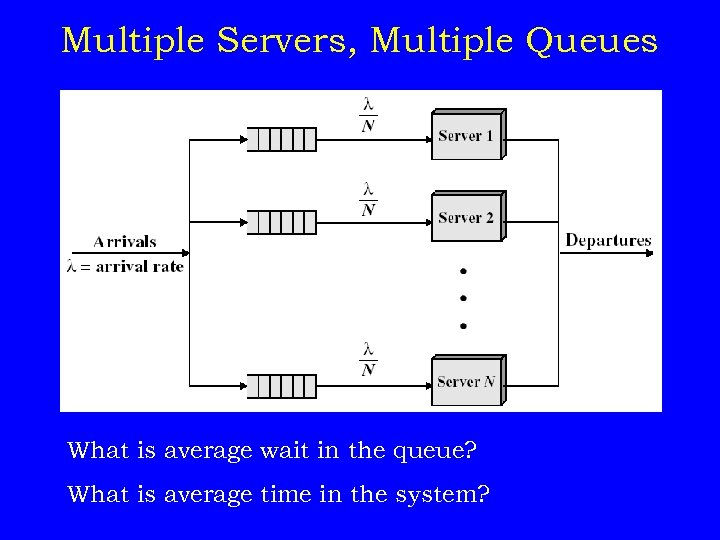Multiple Servers, Multiple Queues What is average wait in the queue? What is average time in the system?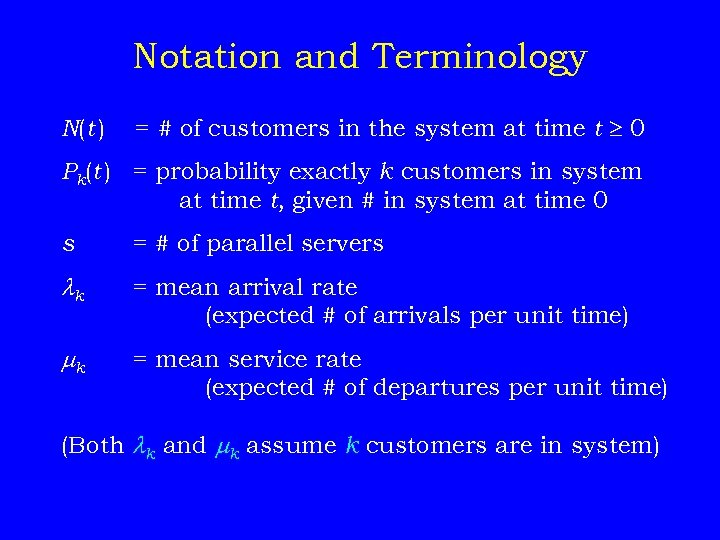Notation and Terminology N(t ) = # of customers in the system at time t 0 Pk(t ) = probability exactly k customers in system at time t, given # in system at time 0 s = # of parallel servers k = mean arrival rate (expected # of arrivals per unit time) k = mean service rate (expected # of departures per unit time) (Both k and k assume k customers are in system)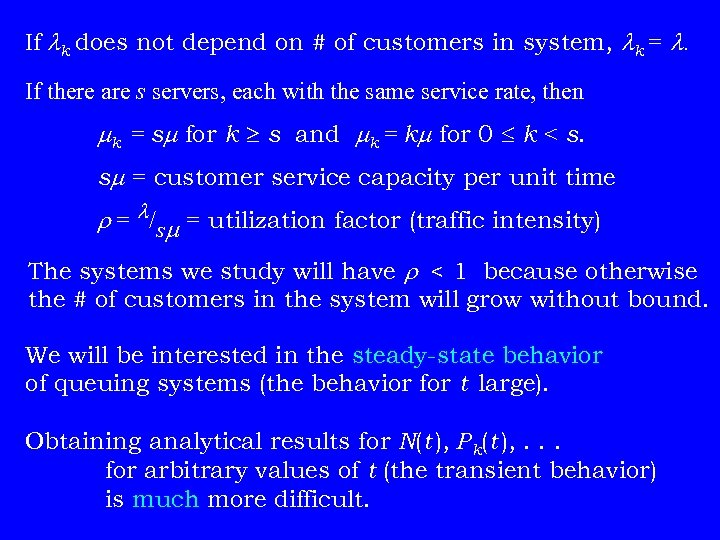If k does not depend on # of customers in system, k = . If there are s servers, each with the same service rate, then k = s for k s and k = k for 0 k < s. s = customer service capacity per unit time r = /s = utilization factor (traffic intensity) The systems we study will have r < 1 because otherwise the # of customers in the system will grow without bound. We will be interested in the steady-state behavior of queuing systems (the behavior for t large). Obtaining analytical results for N(t ), Pk(t ), . . . for arbitrary values of t (the transient behavior) is much more difficult.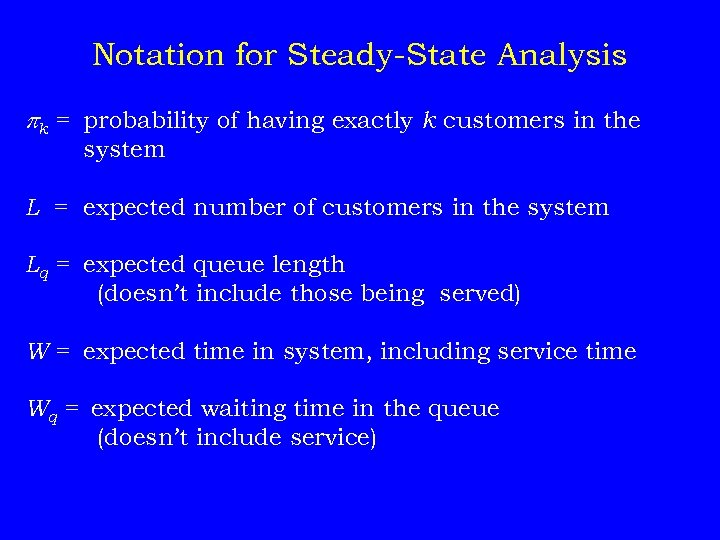Notation for Steady-State Analysis pk = probability of having exactly k customers in the system L = expected number of customers in the system Lq = expected queue length (doesn’t include those being served) W = expected time in system, including service time Wq = expected waiting time in the queue (doesn’t include service)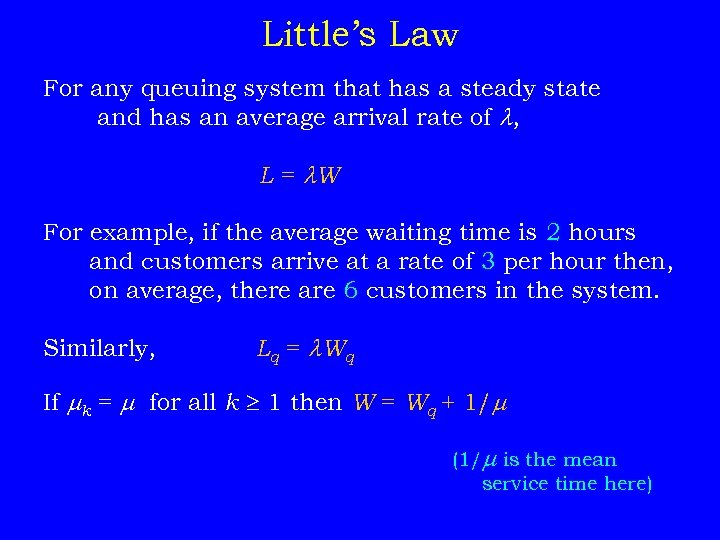Little’s Law For any queuing system that has a steady state and has an average arrival rate of , L = W For example, if the average waiting time is 2 hours and customers arrive at a rate of 3 per hour then, on average, there are 6 customers in the system. Similarly, Lq = W q If k = for all k 1 then W = Wq + 1/ (1/ is the mean service time here)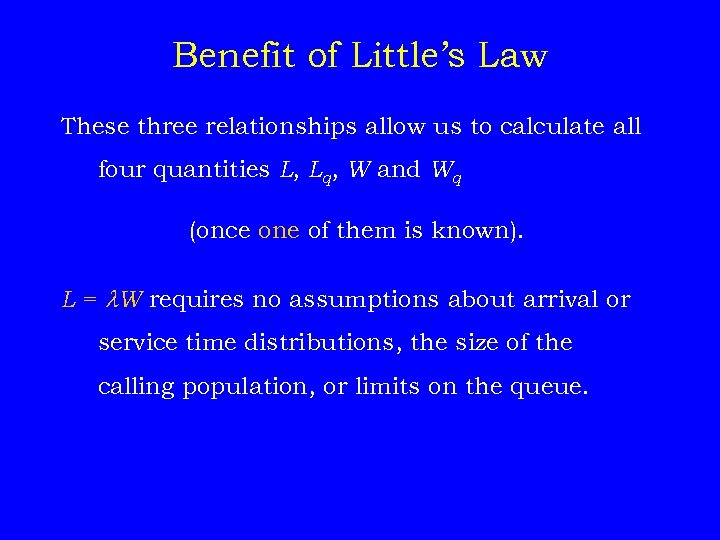Benefit of Little’s Law These three relationships allow us to calculate all four quantities L, Lq, W and Wq (once one of them is known). L = W requires no assumptions about arrival or service time distributions, the size of the calling population, or limits on the queue.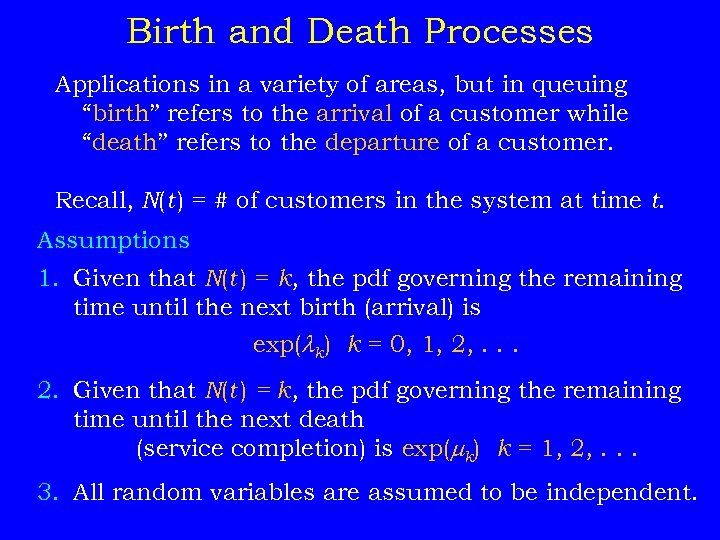Birth and Death Processes Applications in a variety of areas, but in queuing “birth” refers to the arrival of a customer while “death” refers to the departure of a customer. Recall, N(t ) = # of customers in the system at time t. Assumptions 1. Given that N(t ) = k, the pdf governing the remaining time until the next birth (arrival) is exp( k) k = 0, 1, 2, . . . 2. Given that N(t ) = k, the pdf governing the remaining time until the next death (service completion) is exp( k) k = 1, 2, . . . 3. All random variables are assumed to be independent.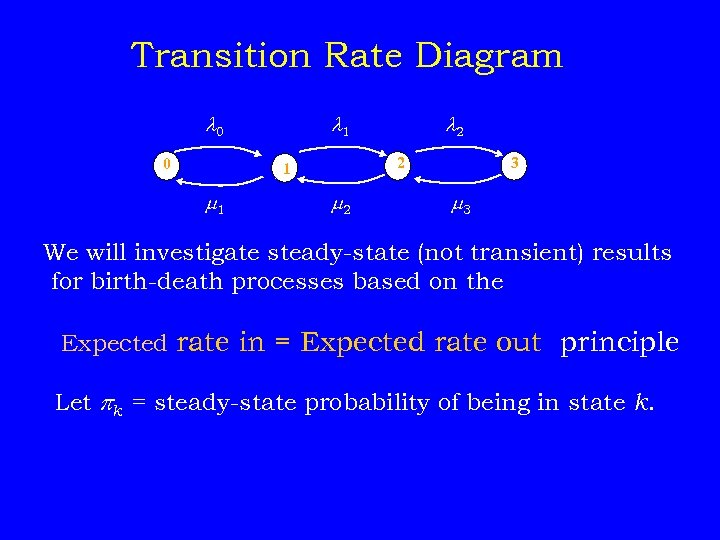Transition Rate Diagram 0 0 1 2 1 1 2 2 3 3 We will investigate steady-state (not transient) results for birth-death processes based on the Expected rate in = Expected rate out principle Let pk = steady-state probability of being in state k.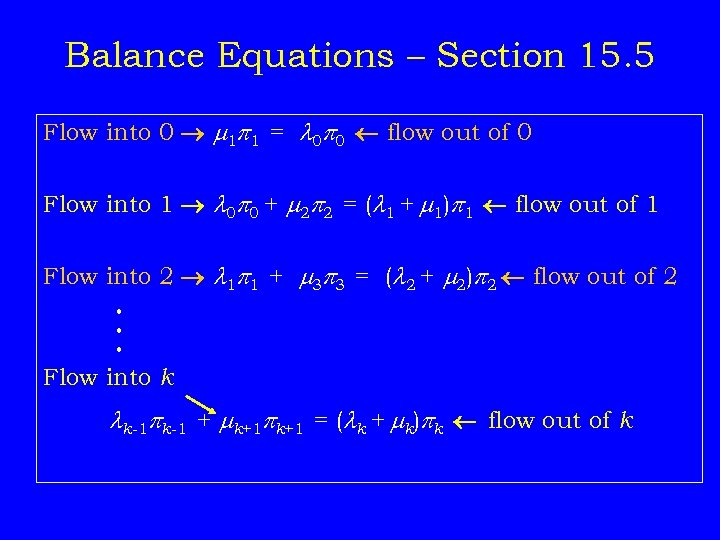Balance Equations – Section 15. 5 Flow into 0 1 p 1 = 0 p 0 flow out of 0 Flow into 1 0 p 0 + 2 p 2 = ( 1 + 1)p 1 flow out of 1 Flow into 2 1 p 1 + 3 p 3 = ( 2 + 2)p 2 flow out of 2 • • • Flow into k k-1 pk-1 + k+1 pk+1 = ( k + k)pk flow out of k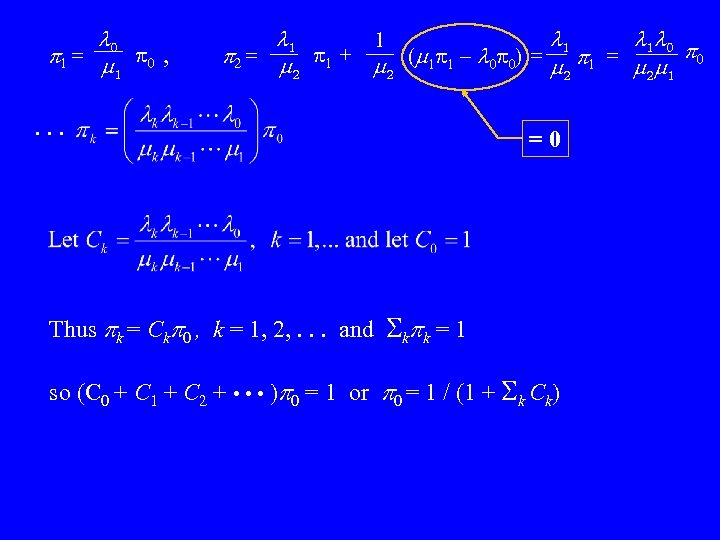0 p 1 = p 0 , 1 1 1 1 0 1 p 2 = p 1 + ( 1 p 1 – 0 p 0) = p 1 = p 0 2 2 1 . . . =0 Thus pk = Ckp 0 , k = 1, 2, . . . and kpk = 1 so (C 0 + C 1 + C 2 + • • • )p 0 = 1 or p 0 = 1 / (1 + k Ck)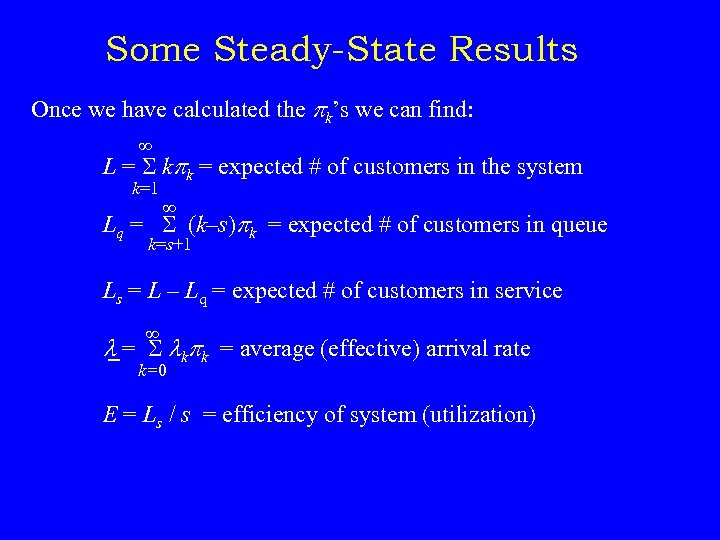Some Steady-State Results Once we have calculated the pk’s we can find: L = kpk = expected # of customers in the system k=1 Lq = (k–s)pk = expected # of customers in queue k=s+1 Ls = L – Lq = expected # of customers in service = kpk = average (effective) arrival rate k=0 E = Ls / s = efficiency of system (utilization)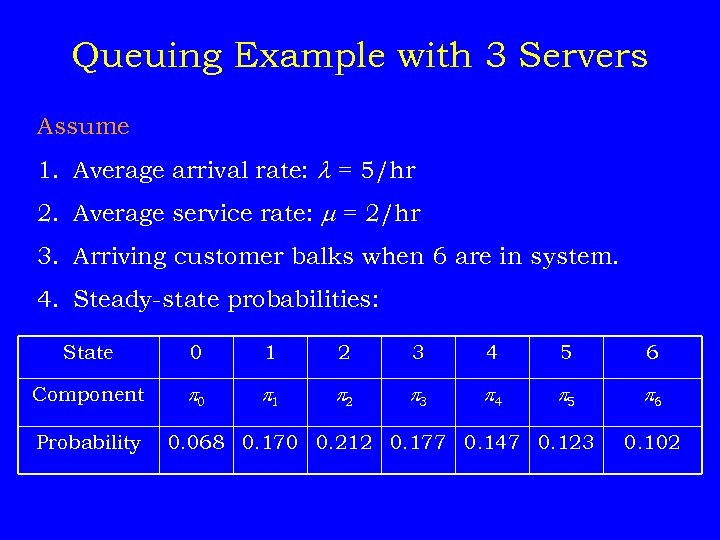Queuing Example with 3 Servers Assume 1. Average arrival rate: = 5/hr 2. Average service rate: = 2/hr 3. Arriving customer balks when 6 are in system. 4. Steady-state probabilities: State 0 1 2 3 4 5 6 Component p 0 p 1 p 2 p 3 p 4 p 5 p 6 Probability 0. 068 0. 170 0. 212 0. 177 0. 147 0. 123 0. 102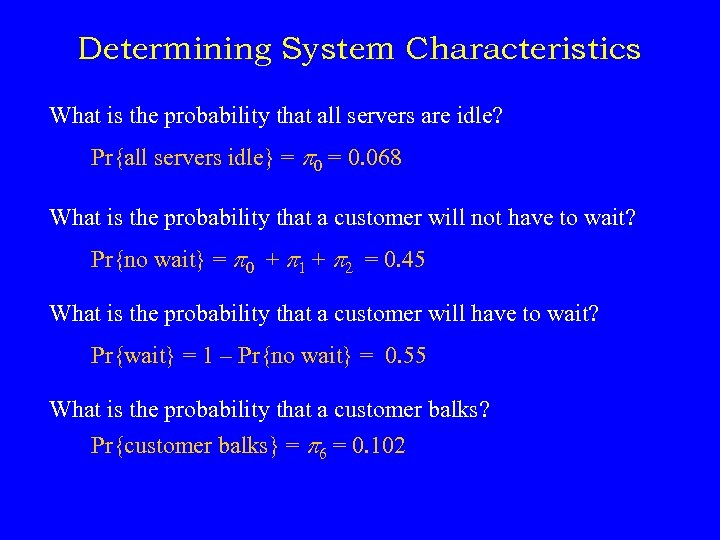Determining System Characteristics What is the probability that all servers are idle? Pr{all servers idle} = p 0 = 0. 068 What is the probability that a customer will not have to wait? Pr{no wait} = p 0 + p 1 + p 2 = 0. 45 What is the probability that a customer will have to wait? Pr{wait} = 1 – Pr{no wait} = 0. 55 What is the probability that a customer balks? Pr{customer balks} = p 6 = 0. 102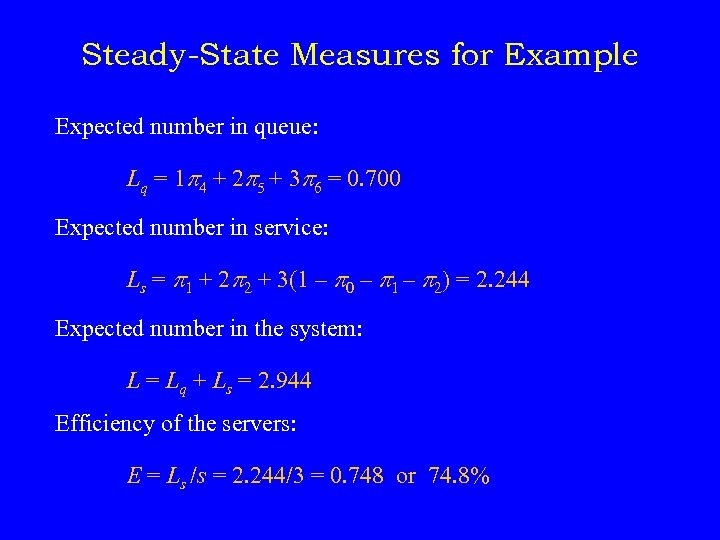Steady-State Measures for Example Expected number in queue: Lq = 1 p 4 + 2 p 5 + 3 p 6 = 0. 700 Expected number in service: Ls = p 1 + 2 p 2 + 3(1 – p 0 – p 1 – p 2) = 2. 244 Expected number in the system: L = Lq + Ls = 2. 944 Efficiency of the servers: E = Ls /s = 2. 244/3 = 0. 748 or 74. 8%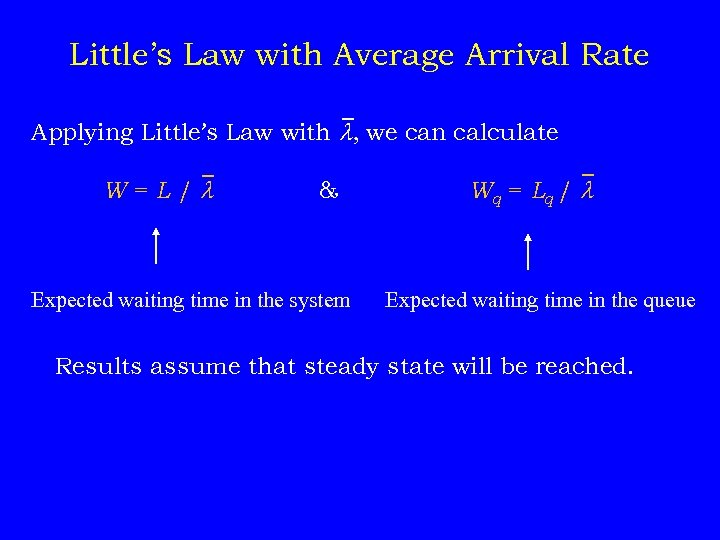Little’s Law with Average Arrival Rate Applying Little’s Law with , we can calculate W=L/ & Expected waiting time in the system W q = Lq / Expected waiting time in the queue Results assume that steady state will be reached.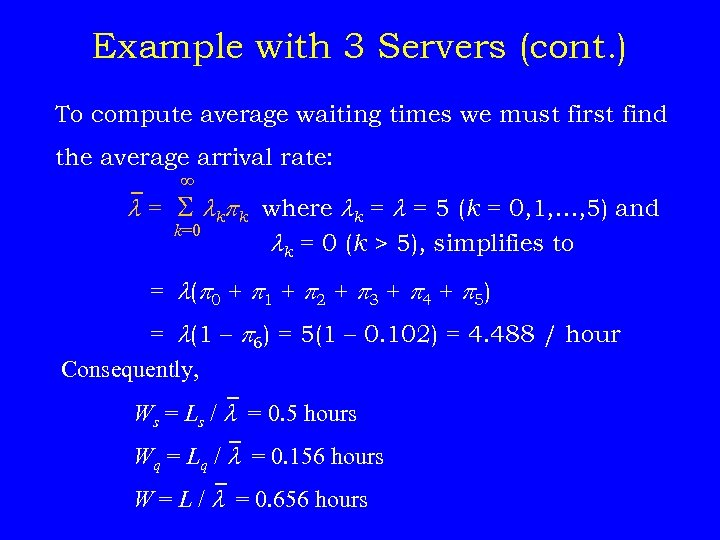Example with 3 Servers (cont. ) To compute average waiting times we must first find the average arrival rate: = kpk where k = = 5 (k = 0, 1, …, 5) and k=0 k = 0 (k > 5), simplifies to = ( p 0 + p 1 + p 2 + p 3 + p 4 + p 5) = (1 – p 6) = 5(1 – 0. 102) = 4. 488 / hour Consequently, Ws = Ls / = 0. 5 hours Wq = Lq / = 0. 156 hours W = L / = 0. 656 hours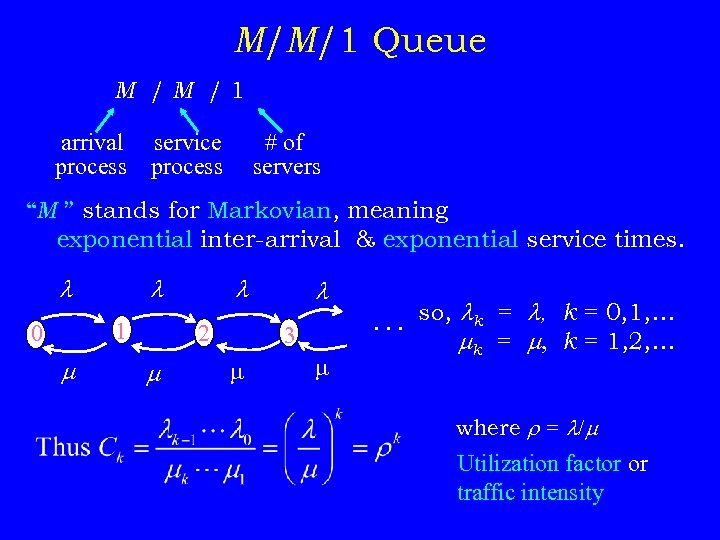M/M/1 Queue M /M /1 arrival process service process # of servers “M ” stands for Markovian, meaning exponential inter-arrival & exponential service times. 1 0 2 3 . . . so, k = , k = 0, 1, … k = , k = 1, 2, … where r = / Utilization factor or traffic intensity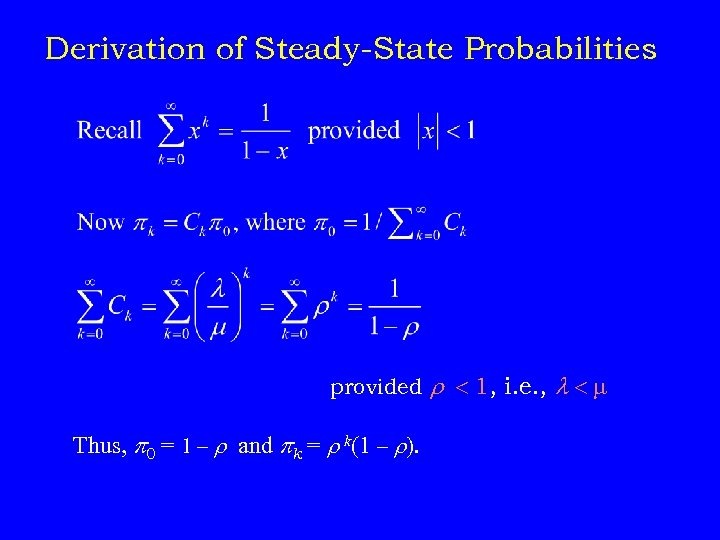Derivation of Steady-State Probabilities provided r 1, i. e. , Thus, p 0 = 1 – r and pk = r k(1 – r).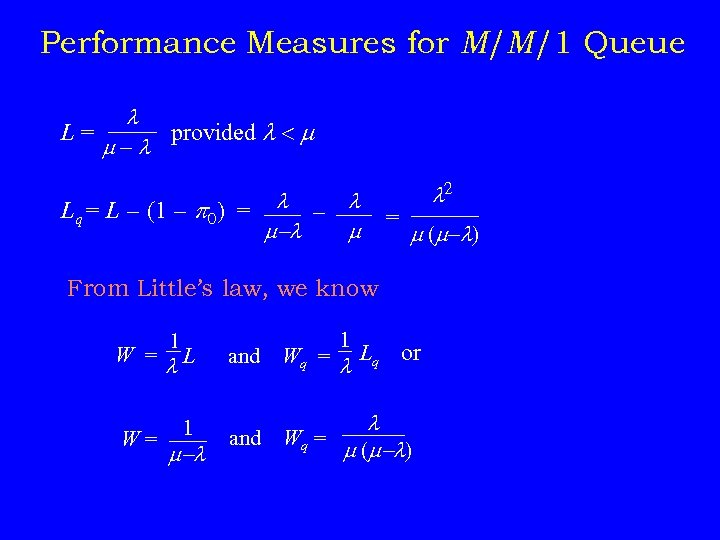Performance Measures for M/M/1 Queue L= provided - Lq = L - (1 - p 0) = - - = 2 ( - ) From Little’s law, we know 1 W = L W= 1 - and 1 Wq = Lq or and Wq = ( - )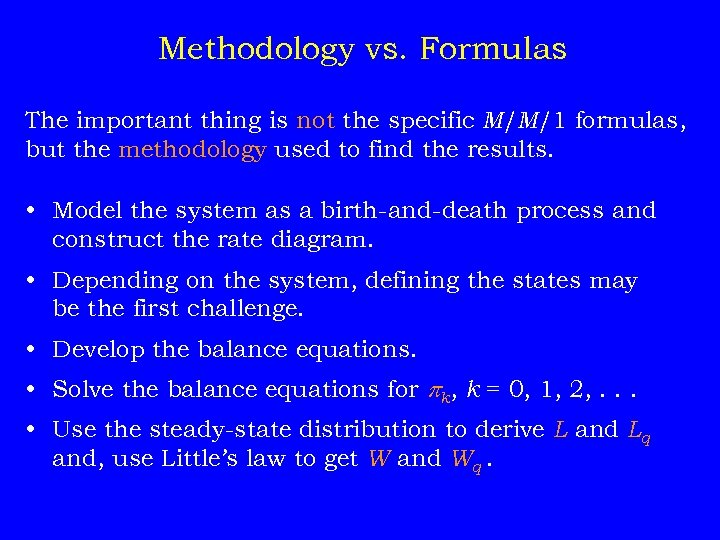Methodology vs. Formulas The important thing is not the specific M/M/1 formulas, but the methodology used to find the results. • Model the system as a birth-and-death process and construct the rate diagram. • Depending on the system, defining the states may be the first challenge. • Develop the balance equations. • Solve the balance equations for pk, k = 0, 1, 2, . . . • Use the steady-state distribution to derive L and Lq and, use Little’s law to get W and Wq.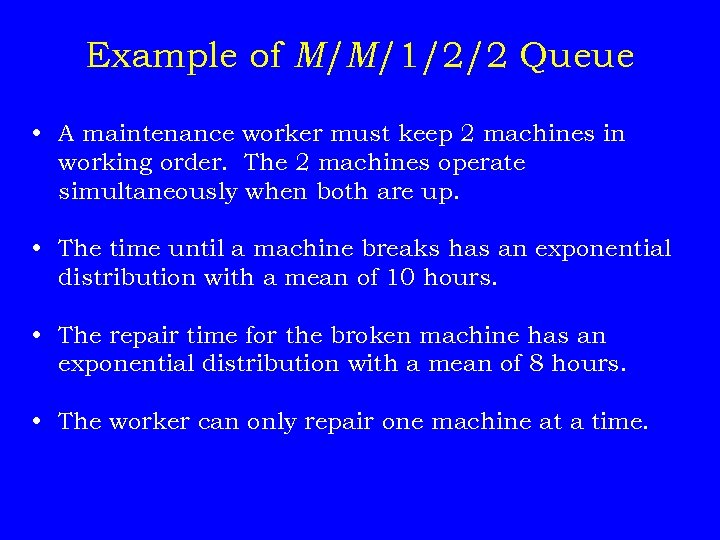Example of M/M/1/2/2 Queue • A maintenance worker must keep 2 machines in working order. The 2 machines operate simultaneously when both are up. • The time until a machine breaks has an exponential distribution with a mean of 10 hours. • The repair time for the broken machine has an exponential distribution with a mean of 8 hours. • The worker can only repair one machine at a time.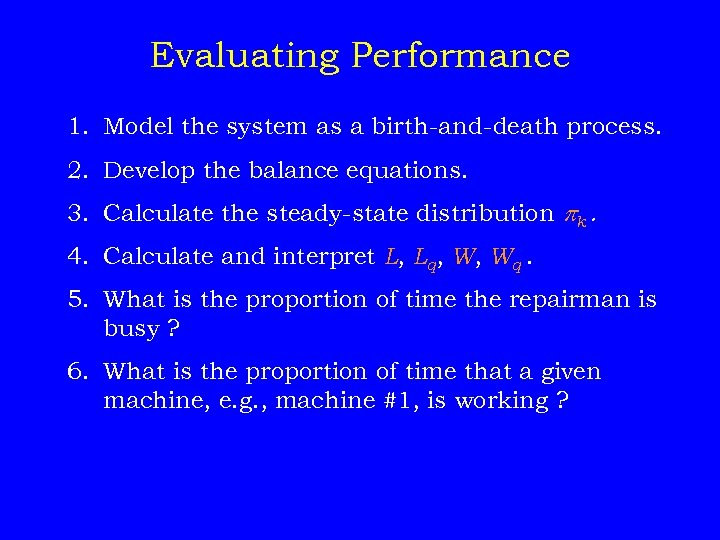Evaluating Performance 1. Model the system as a birth-and-death process. 2. Develop the balance equations. 3. Calculate the steady-state distribution pk. 4. Calculate and interpret L, Lq, W, Wq. 5. What is the proportion of time the repairman is busy ? 6. What is the proportion of time that a given machine, e. g. , machine #1, is working ?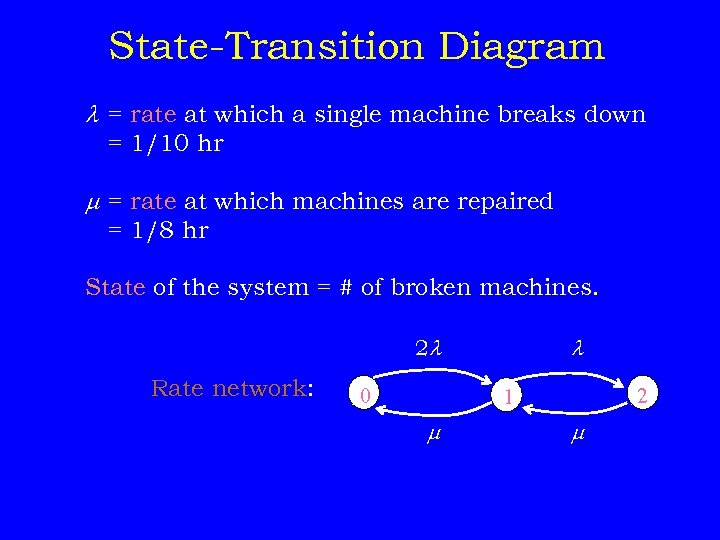State-Transition Diagram = rate at which a single machine breaks down = 1/10 hr = rate at which machines are repaired = 1/8 hr State of the system = # of broken machines. 2 Rate network: 0 2 2 1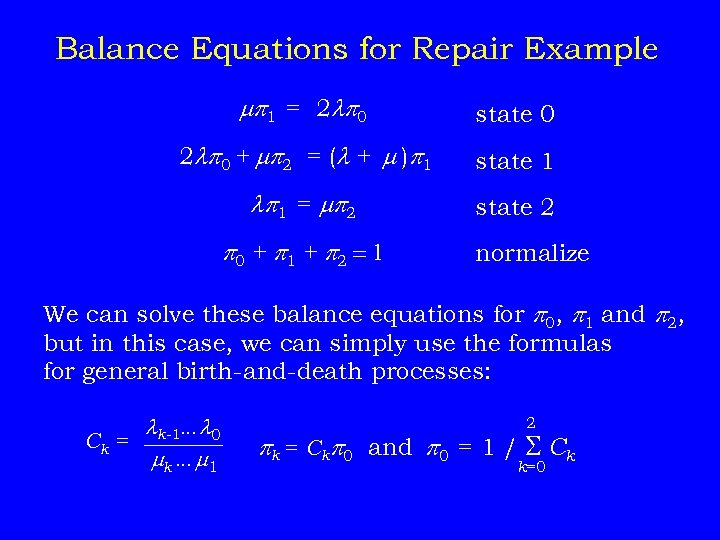Balance Equations for Repair Example p 1 = 2 p 0 state 0 2 p 0 + p 2 = ( + )p 1 state 1 p 1 = p 2 state 2 p 0 + p 1 + p 2 = 1 normalize We can solve these balance equations for p 0, p 1 and p 2, but in this case, we can simply use the formulas for general birth-and-death processes: k-1… 0 Ck = k … 1 2 pk = Ckp 0 and p 0 = 1 / Ck k=0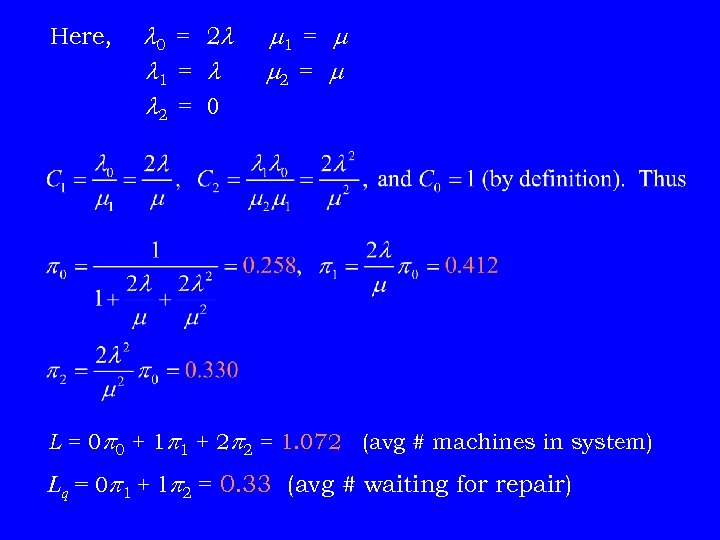Here, 0 = 2 1 = 2 = 0 1 = 2 = L = 0 p 0 + 1 p 1 + 2 p 2 = 1. 072 (avg # machines in system) Lq = 0 p 1 + 1 p 2 = 0. 33 (avg # waiting for repair)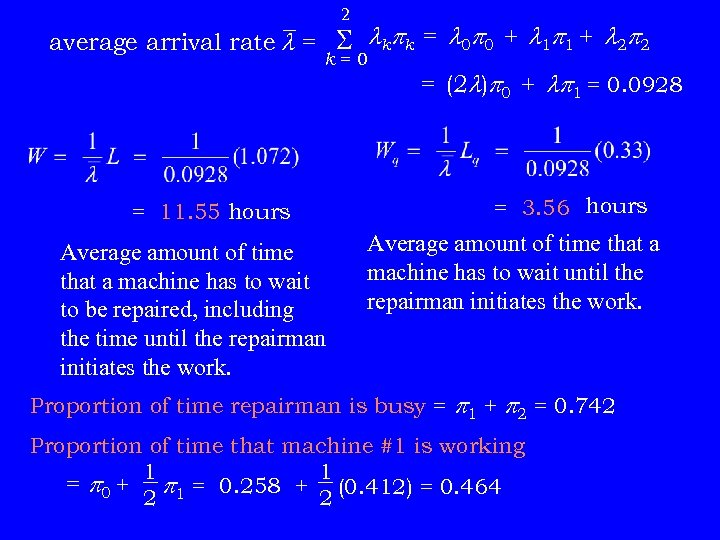2 average arrival rate = kpk = 0 p 0 + 1 p 1 + 2 p 2 k=0 = 11. 55 hours Average amount of time that a machine has to wait to be repaired, including the time until the repairman initiates the work. = (2 )p 0 + p 1 = 0. 0928 = 3. 56 hours Average amount of time that a machine has to wait until the repairman initiates the work. Proportion of time repairman is busy = p 1 + p 2 = 0. 742 Proportion of time that machine #1 is working 1 1 = p 0 + p 1 = 0. 258 + (0. 412) = 0. 464 2 2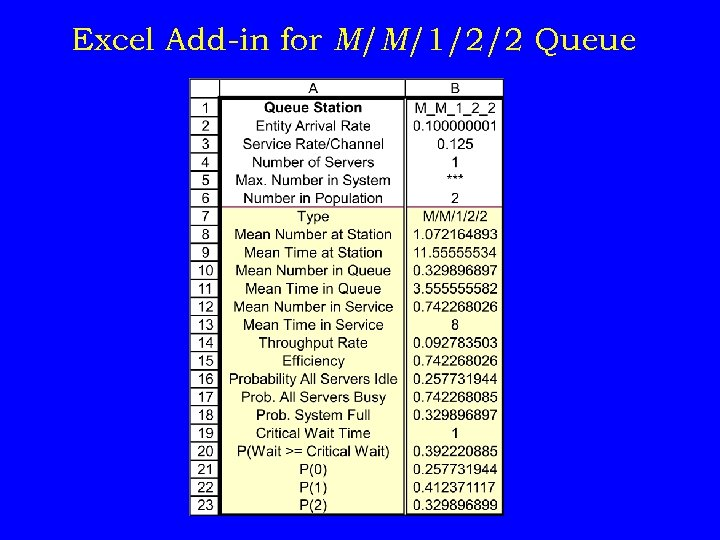Excel Add-in for M/M/1/2/2 Queue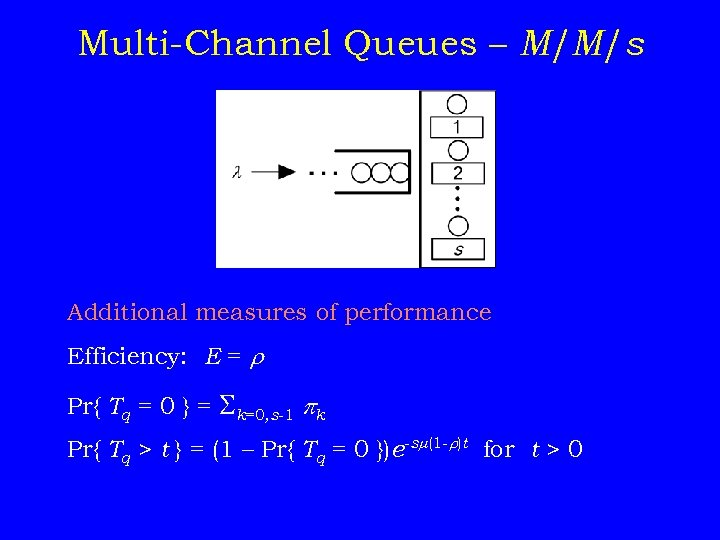Multi-Channel Queues – M/M/s Additional measures of performance Efficiency: E = r Pr{ Tq = 0 } = k=0, s-1 pk Pr{ Tq > t } = (1 – Pr{ Tq = 0 })e-s (1 -r)t for t > 0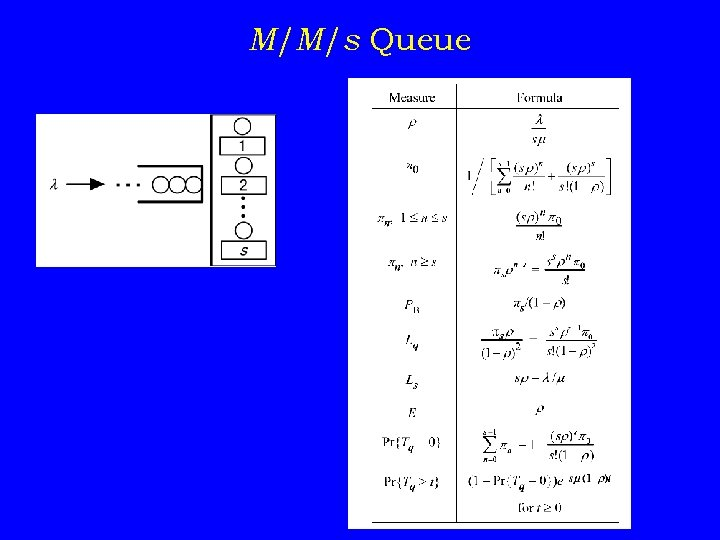M/M/s Queue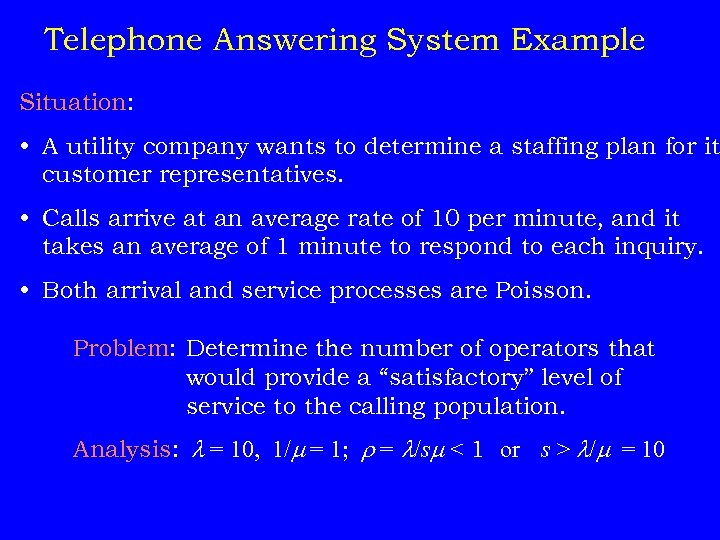Telephone Answering System Example Situation: • A utility company wants to determine a staffing plan for it customer representatives. • Calls arrive at an average rate of 10 per minute, and it takes an average of 1 minute to respond to each inquiry. • Both arrival and service processes are Poisson. Problem: Determine the number of operators that would provide a “satisfactory” level of service to the calling population. Analysis: = 10, 1/ = 1; r = /s < 1 or s > / = 10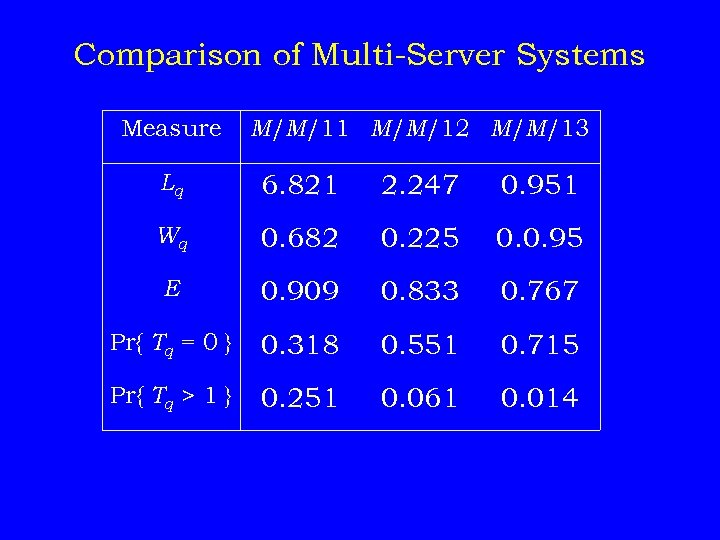Comparison of Multi-Server Systems Measure M/M/11 M/M/12 M/M/13 Lq 6. 821 2. 247 0. 951 Wq 0. 682 0. 225 0. 0. 95 E 0. 909 0. 833 0. 767 Pr{ Tq = 0 } 0. 318 0. 551 0. 715 Pr{ Tq > 1 } 0. 251 0. 061 0. 014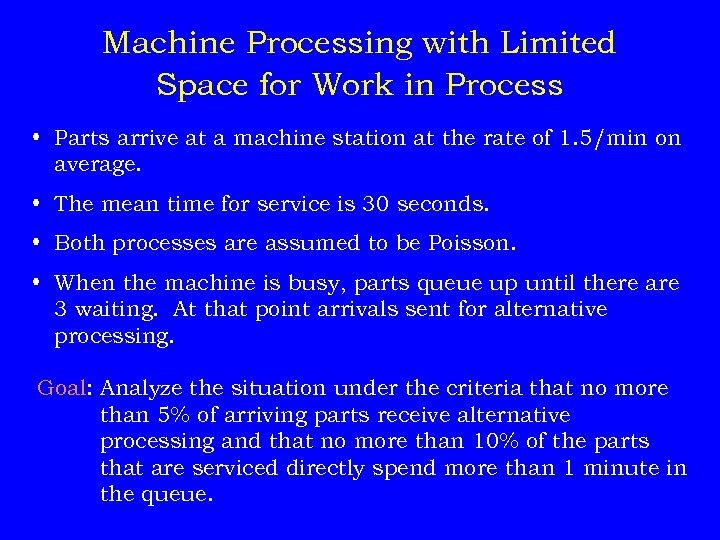Machine Processing with Limited Space for Work in Process • Parts arrive at a machine station at the rate of 1. 5/min on average. • The mean time for service is 30 seconds. • Both processes are assumed to be Poisson. • When the machine is busy, parts queue up until there are 3 waiting. At that point arrivals sent for alternative processing. Goal: Analyze the situation under the criteria that no more than 5% of arriving parts receive alternative processing and that no more than 10% of the parts that are serviced directly spend more than 1 minute in the queue.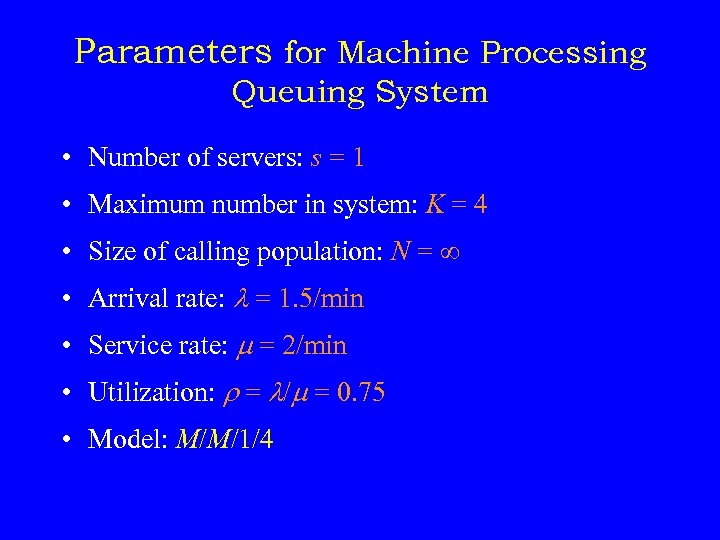Parameters for Machine Processing Queuing System • Number of servers: s = 1 • Maximum number in system: K = 4 • Size of calling population: N = • Arrival rate: = 1. 5/min • Service rate: = 2/min • Utilization: r = / = 0. 75 • Model: M/M/1/4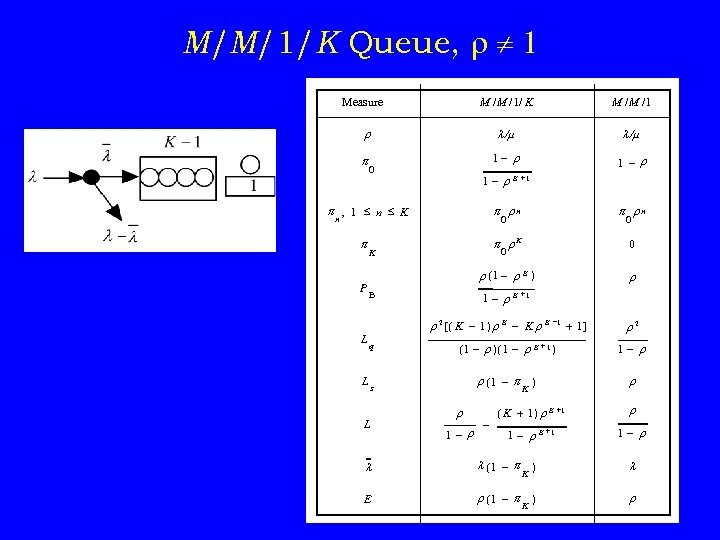M/M/1/K Queue, r 1 M /M /1/ K Measure M /M /1 r / / p 1 - r 1 – r 0 1 - r K +1 p , 1 n K n p rn p p r. K 0 r (1 - r K ) r P L 0 0 0 K B 1 - r. K +1 r 2 [( K - 1 ) r K - K r K -1 + 1 ] q r 2 (1 - r )(1 - r K + 1 ) 1 - r r (1 – p ) K r Ls L r 1 – r – ( K + 1) r K + 1 1 - r K +1 r 1 - r (1 – p ) K E r (1 – p ) K r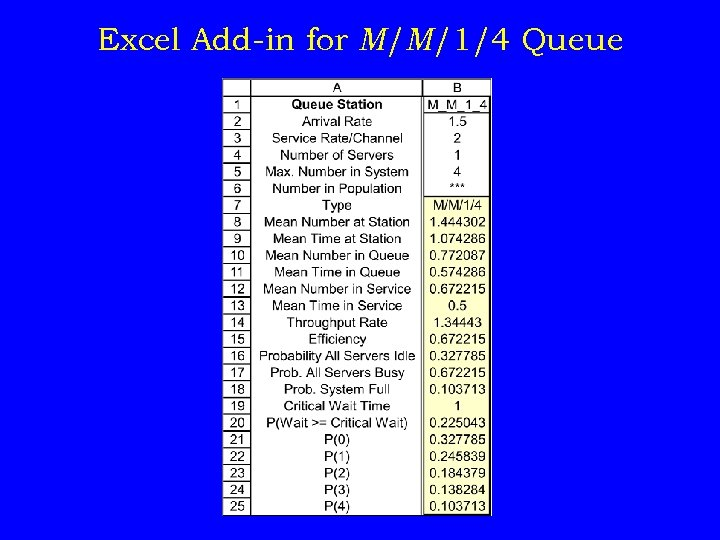Excel Add-in for M/M/1/4 Queue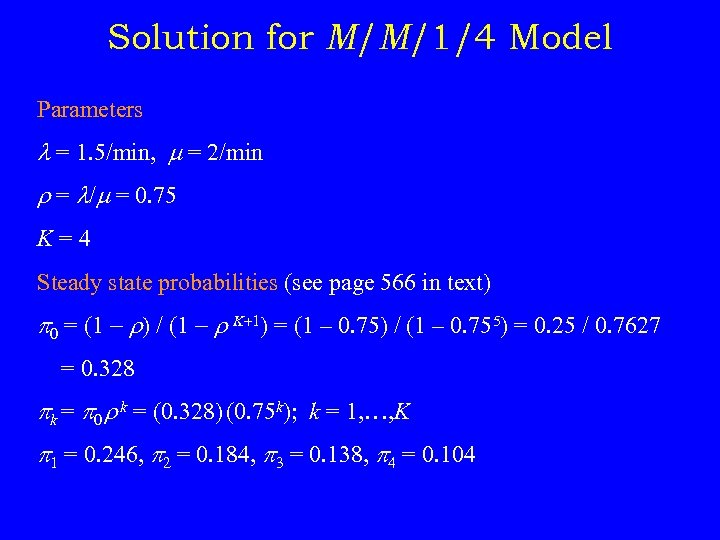Solution for M/M/1/4 Model Parameters = 1. 5/min, = 2/min r = / = 0. 75 K=4 Steady state probabilities (see page 566 in text) p 0 = (1 - r) / (1 - r K+1) = (1 – 0. 75) / (1 – 0. 755) = 0. 25 / 0. 7627 = 0. 328 pk = p 0 r k = (0. 328) (0. 75 k); k = 1, …, K p 1 = 0. 246, p 2 = 0. 184, p 3 = 0. 138, p 4 = 0. 104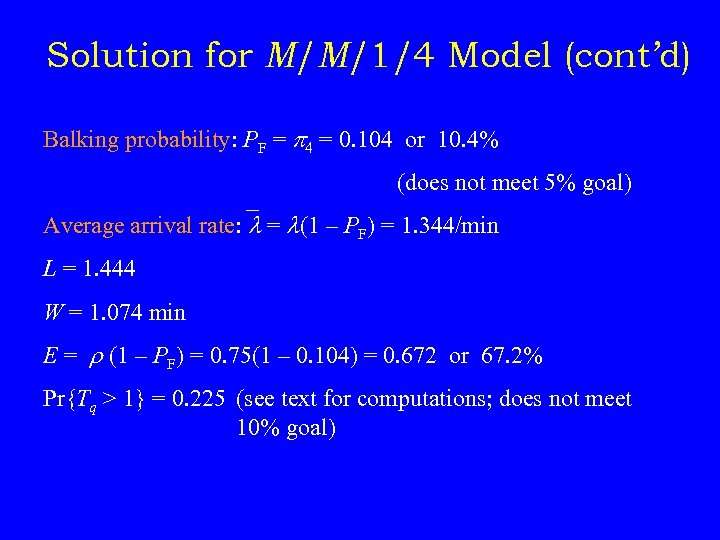Solution for M/M/1/4 Model (cont’d) Balking probability: PF = p 4 = 0. 104 or 10. 4% (does not meet 5% goal) Average arrival rate: = (1 – PF) = 1. 344/min L = 1. 444 W = 1. 074 min E = r (1 – PF) = 0. 75(1 – 0. 104) = 0. 672 or 67. 2% Pr{Tq > 1} = 0. 225 (see text for computations; does not meet 10% goal)Add Second Machine – M/M/2/5 Model New results PF = 0. 0068, = (1 – p 5) = 1. 49 L = 0. 85, W = 0. 57 min E = 37. 2% Pr{Tq > 1} = 0. 049 or 4. 9% This solution meets our original goals with the percentage of balking parts now less than 1% and the probability of a wait time greater than 1 minute less than 5%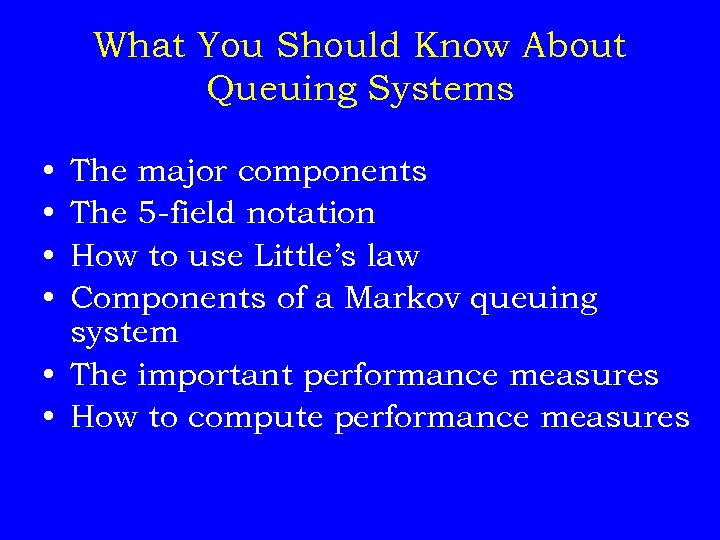What You Should Know About Queuing Systems • • The major components The 5 -field notation How to use Little’s law Components of a Markov queuing system • The important performance measures • How to compute performance measures